# HBSE 8th Class Maths Solutions Chapter 3 Understanding Quadrilaterals InText Questions

Haryana State Board HBSE 8th Class Maths Solutions Chapter 3 Understanding Quadrilaterals InText Questions and Answers.

## Haryana Board 8th Class Maths Solutions Chapter 3 Understanding Quadrilaterals InText Questions

Do This (Page 40-41)

Question 1.
Take any quadrilateral, say ABCD (in figure). Divide it into two triangles, by drawing a diagonal. You get six angles 1,2, 3, 4, 5 and 6.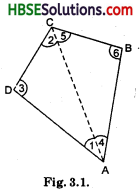Use the angle-sum property of a triangle and argue how the sum of the measures of ∠A, ∠B, ∠C and ∠D amounts to 180°+ 180° = 360°.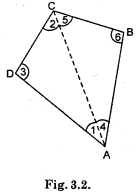Solution:
In △ACD
∠DAC + ∠ACD + ∠ADC = 180° (The angle sum property of a triangle)
or ∠1+ ∠2 + ∠3 = 180° …….(i)
In △ABC,
∠CAB + ∠ACB + ∠ABC = 180°
∠4 + ∠5 + ∠6 = 180° …….(ii)
Adding (i) and (ii) we get
(∠1 + ∠4) + (∠2 + ∠ 5) + ∠3 + ∠6 = 360°
or ∠DAB + ∠DCB + ∠ADC + ∠ABC = 360°
∴ ∠A + ∠B + ∠C + ∠D = 360°.

Question 2.
Take four congruent card-board copies of any quadrilateral ABQD, with angles as shown in Fig. 3.3(i). Arrange the copies as shown in the figure where angles ∠1, ∠2, ∠3, ∠4 meet At a point [Fig. 3.3(ii)]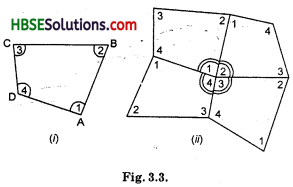What can you say about the sum of the angles ∠1, ∠2, ∠3 and ∠4 ?
Solution:
The sum of the measures of the four angles of a quadrilateral is you may arrive at this result in several other ways also.
∠1 + ∠2 + ∠3 + ∠4 = 360°
The sum of the measures of the four angles of a quadrilateral is 360°.Question 3.
As before consider quadrilateral ABCD in Fig. 3.4.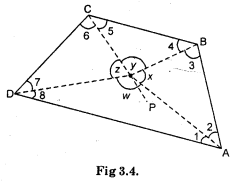Let P be any point in its interior. Join P to vertices A, B, C and D. In the figure, consider △PAB. From this we see x = 180° – m∠2 – m∠3; similarly from △PBC, y = 180° – m∠4 – m∠5, from △SPCD, z = 180° – m∠6 – m∠7 and from △PDA, w = 180°- m∠8- m∠1. Use this to find the total measure m∠l + m∠2 + ………. + m∠8, does it help you to arrive at the result ?
Remember:
∠x + ∠y + ∠z + ∠W = 360°
Solution:
In △PAB, ∠x = 180° – m∠2 -m∠3 …(i)
[The angle-sum property of a triangle]
In △PBC, ∠y = 180° — m∠4 – m∠5 ….(ii)
In △PCD, ∠z = 180° – m∠6 – m∠7 …(iü)

Question 4.
These quadrilaterals were convex. What would happen if the quadrilateral is not convex ? Consider quadrilateral ABCD. Split it into two triangles and find the sum of the interior angles.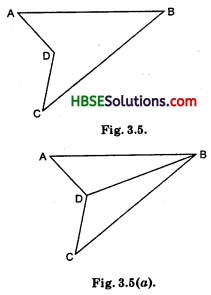Solution:
In △ABD,
In △BDC,
∠BDC + ∠DBC + ∠BCD = 180° …(ii)
(∠ABD + ∠DBC) + (∠ADB + ∠CBD) + (∠DAB + ∠DCB) = 360°
∠ABC + ∠ADC + ∠DAB + ∠DCB = 360°.Try These (Page 43)

Take a regular hexagon Fig. 3.12.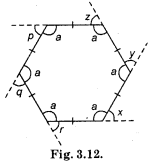Question 1.
What is the sum of the measures of its exterior angles x, y, z, p q, r ?
Solution:
(∠a + ∠r) + (∠a + ∠x) + (∠a + ∠y) + (∠a + ∠z) + (∠a + ∠p) + (∠a + ∠q) = 6 × 180°
[∵ ∠a + ∠r, ∠a + ∠x ……. (∠a + ∠q formed linear pair]
or ∠a + ∠a + ∠a + ∠a + ∠a + ∠a + (∠r + ∠x + ∠y + ∠z + ∠p + ∠q) = 1080°
or 6∠a + 360° = 1080°
[Sum of exterior angles of a polygon]
or 6∠a = 1080-360°
= 720°
∴ ∠a = $$\frac{720}{6}$$ = 120°
Therefore sum of its exterior angle
=∠q + ∠r + ∠x + ∠y + ∠z + ∠p
=(180 – 120) × 6
= 360°.

Question 2.
Is x = y = z = p = q = r ? Why ?
Solution:
Yes, ∠a = 120°
∴ ∠a + ∠r =∠a + ∠x = ∠a + ∠y
= ∠a + ∠z = ∠a + ∠p
= ∠a + ∠q [Linear pair]
But ∠a = 120°
∴ ∠r = ∠x = ∠y
= ∠z = ∠p = ∠q
= 180 – 120° = 60°.Question 3.
What is the measure of each ?
(i) Exterior angle
(ii) Interior angle.
Solution:
(i) The measure of each exterior angle = $$\frac{360}{6}$$ = 60°
(ii) The measure of each interior angle = a + 60° = 180°
∴ a = 120°.

Question 4.
Repeat this activity for the cases of
(i) a regular octagon
(ii) a regular 20-gon
Solution:
The exterior angle of a regular octagon
= $$\frac{360}{8}$$ = 45°
The exterior angle of a regular 20-gon
= $$\frac{360}{20}$$ = 18°
The interior angle of a regular octagon
= 180° – 45° = 135°
The interior angle of a regular 20-gon
= 180° – 18° = 162°.Try These (Page 43)

Question 1.
Take two identical set squares with angles 30° – 60° – 90° and place them adjacently to form a parallelogram as shewn in Fig. 3.20. Does this help you to verify the above property?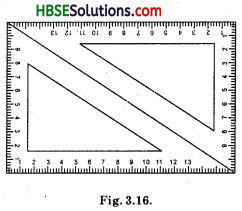Solution:
we know that a parallelogram is a quadrilateral whose opposite sides are parallel.
From figure,
∠D = 60° + 30° = 90°
∠A = 90°
∠C = 90°
Similarly, ∠B = 30° + 60° = 90°
From figure $$\overline{\mathrm{AB}}$$ and $$\overline{\mathrm{CD}}$$ are opposite sides and $$\overline{\mathrm{AD}}$$ and $$\overline{\mathrm{BC}}$$ are another opposite side.
∠A and ∠C are a pair of opposite angles.
∠B and ∠C are another pair of opposite angles.
$$\overline{\mathrm{AB}}$$ and $$\overline{\mathrm{BC}}$$ are adjacent sides. This means, one of the sides starts when the other sides.
Similarly, $$\overline{\mathrm{BC}}$$ and $$\overline{\mathrm{CD}}$$ are adjacent sides.
Thus, the property of the parallelogram opposite sides are equal and parallel knd their opposite angles are equal.
Hence, this figure is parallelogram or rectangle and verified.

Try These (Page 48)

Question 1.
Take two identical 30° – 60° – 90° set-squares and form a parallelogram as before. Does the figure obtained help you to confirm the above property?
Solution:
From figure
∠1 = ∠2 + 30° = alternate angle
∠3 = ∠4 = 60° alternate angle
Hence ∠B = ∠D = 90°
When AB and CD are parallel then its angles ∠1 and ∠2 are alternate angles and mutually equal.
or, if alternate angles are equal then opposite side AB and CD are parallel.
According to property of parallelogram opposite sides and opposite angles are equal.
Hence, ∠A = ∠C = 90° and ∠B = ∠D = 90°
and AB = CD andBC = AD.
Hence this is verified.